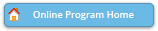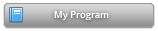#### Abstract Details

 Activity Number: 271 - Statistical Analysis of Complex Imaging Data Type: Invited Date/Time: Tuesday, July 30, 2019 : 8:30 AM to 10:20 AM Sponsor: Section on Statistics in Imaging Abstract #308079 Presentation Title: Tensor Approximation for Partial Quantile Regression in Functional Linear Models Author(s): Dengdeng Yu* Companies: University of Toronto Keywords: Abstract: We consider the estimation problem in functional linear quantile regression in which the dependent variable is a scalar while the covariate is a function. The conditional quantile of response is modelled as a linear functional of the covariate. We are interested in the way of efficiently and effectively extracting the basis for estimating functional coefficients. There are two common approaches. One is to use the functional principal components. The other one is to use functional partial quantile basis which is just an extension of partial least squares into quantile regression. As a supervised method, partial quantile method is superior to unsupervised PCA method under certain circumstance. In this talk, we propose to use the partial quantile regression and its tensor approximation to estimate the functional effect in functional linear quantile regression model. Asymptotic properties have been studied to show the theoretical performance while both a simulation and a neuroimaging data example are investigated and some interesting findings are discovered.

Authors who are presenting talks have a * after their name.